Next: 3.1.4 The Lattice Heat Up: 3.1 Sets of Partial Previous: 3.1.2 The Drift-Diffusion Transport

## 3.1.3 The Hydrodynamic Transport Model

In the hydrodynamic transport model, carrier temperatures are assumed to be different from the lattice temperature. The basic equations (3.2) through (3.4) are augmented by energy balance equations which determine the carrier temperatures. The current relations take the form

 Jn = q .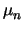. n .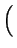grad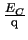-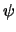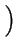+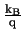.. grad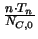, (3.7)

 Jp = q .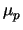. p .grad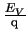--.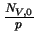. grad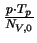. (3.8)

The energy balance equations state conservation of the average carrier energies. In terms of the carrier temperatures, Tn and Tp, they can be written as
 div Sn = grad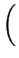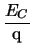-. Jn -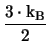.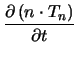+ R . Tn + n .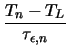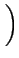(3.9) div Sp = grad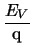-. Jp -.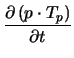+ R . Tp + p .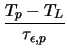. (3.10)

Here,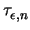and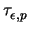denote the energy relaxation times, while Sn and Sp are the energy fluxes.
 Sn = -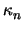. grad Tn -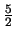.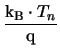. Jn (3.11) Sp = -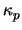. grad Tp +.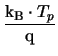. Jp (3.12)

The thermal conductivities,and, are assumed to obey a generalized Wiedemann-Franz law .=+ cn.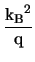. Tn .. n (3.13)=+ cp.. Tp .. p (3.14)Next: 3.1.4 The Lattice Heat Up: 3.1 Sets of Partial Previous: 3.1.2 The Drift-Diffusion Transport
Tibor Grasser
1999-05-31